# Cauchy Schwarz inequality

The Cauchy inequality for finite sums of real numbers is the inequality \begin{equation} \left(\sum_{k=1}^n a_k b_k\right)^2\leq \sum_{k=1}^n a_k^2 \sum_{k=1}^n b_k^2 . \end{equation} Proved by A.L. Cauchy (1821); the analogue for integrals is known as the Bunyakovskii inequality.

The Cauchy inequality is also the name used for an inequality for the modulus $|f^{(k)}(a)|$ of a derivative of a regular analytic function $f(z)$ at a fixed point $a$ of the complex plane $C$, or for the modulus $|c_k|$ of the coefficients of the power series expansion of $f(z)$, \begin{equation} f(z)=\sum_{k=0}^\infty c_k (z-a)^k . \end{equation} These inequalities are(*)

where $r$ is the radius of any disc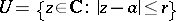on which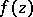is regular, and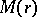is the maximum modulus of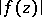on the circle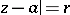. The inequalities (*) occur in the work of A.L. Cauchy (see e.g. ). They directly imply the Cauchy–Hadamard inequality (see ):where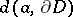is the distance from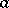to the boundaryof the domain of holomorphy of. In particular, ifis an entire function, then at any point,For a holomorphic functionof several complex variables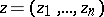,, the Cauchy inequalities are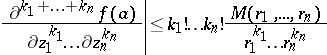orwhere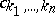are the coefficients of the power series expansion of: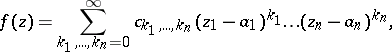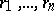are the radii of a polydisc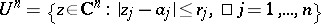on whichis holomorphic, and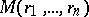is the maximum ofon the distinguished boundary of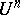.

For references see Cauchy–Hadamard theorem.

The distinguished boundary of a polydisc $U^n$ as above is the set.